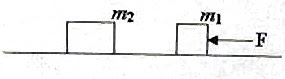## Pages

`“Life is like riding a bicycle.  To keep your balance you must keep moving.”–Albert Einstein`

## Sunday, October 19, 2008

### AP Physics B & C – Practice Questions (MCQ) on Conservation of Linear Momentum

In the post dted 24th September 2008 we had discussed the essential points to be remembered under Newton’s laws of motion. It was then stated that we will discuss the conservation of momentum separately. Today we will discuss some typical multiple choice questions involving the law of conservation of linear momentum.

As you know, Newton’s second law and third law lead to the law of conservation of momentum: The total momentum of an isolated system of interacting particles is conserved.

By isolated system you mean that the system is not acted on by external forces. There may be internal forces by which pairs of particles in the system interact thereby changing their individual momentum. But since the mutual forces for each pair are equal and opposite, the changes in momentum cancel in pairs and the total momentum remains unchanged. A typical case usually used to illustrate the law of conservation of momentum is the recoil of a gun when a bullet is fired. Initially, the bullet and the gun are at rest and hence the momentum of the system (of bullet and gun) is zero. On firing, the bullet moves forward because of the internal forces developed in the system. Since the final momentum of the system has to be zero, the forward momentum of the bullet has to be equal in magnitude to the backward (recoil) momentum of the gun at the instant of firing. If m and M represent the masses of the bullet and the gun and v and V the magnitudes of their velocities respectively, we have

mv + MV = 0

This gives the recoil velocity of the gun: V = mv/M

Now consider the following questions:

(1) A hose-pipe delivering water at the rate of 2.5 litre per second is held horizontally by a fire man. If the rate of flow of water is increased to 5 litre per second by increasing the speed of water, the fire man has to

(a) push forward with the same force as before

(b) push forward twice as hard

(c) push forward four times as hard

(d) push forward with half the force

(e) exert no horizontal force in both cases

Since the water jet has a forward momentum, the hose-pipe should have a backward momentum of the same magnitude (similar to the recoil momentum of a gun firing a bullet). The fire man has to exert a force in the direction of the water jet to hold the hose-pipe in position. When the rate of flow is doubled by increasing the speed of warer, the momentum imparted to the water jet per second is made four times sine the mass and velocity are doubled. Therefore, the fire man has to push forward four times as hard.

(2) A horizontal force F acts for a small time ∆t on an object of mass m1 initially at rest on a smooth horizontal surface (fig.). After the force has ceased, the object gets attached to another stationary object of mass m2 . What is the common velocity of the two objects moving together?(a) Zero

(b) F∆t m1/(m1+ m2)

(c) Fm1/(m1+ m2)

(d) F∆t m2/(m1+ m2)

(e) F∆t/(m1+ m2)

The impulse received by the object of mass m1 is F∆t. The momentum of this object therefore becomes F∆t. [Note that impulse is equal to the change in momentum. Since the mass m1 is initially at rest, its momentum becomes F∆t].

The initial momentum of the system (made of m1 and m2) is thus F∆t. The final momentum also is F∆t since the force has ceased. Therefore, we have

F∆t = (m1+ m2)v where ‘v’ is the common velocity of m1 and m2. This gives

v = F∆t/(m1+ m2)

(3) A shell fired from a cannon with velocity v at an angle of 45º with the horizontal explodes into two parts of equal mass at the highest point of its trajectory. One of the pieces retraces its path. What will be the speed of the other piece immediately after the explosion?

(a) 3v/2

(b) 3v/√2

(c) v/2

(d) v/√2

(e) 2v

At the highest point, the projectile (shell) has horizontal momentum only which is mvcosθ where m is the mass of the shell and θ is the angle of projection (45º here). Thus just before the explosion, the shell has momentum equal to mvcos45º = mv/√2

Since the total momentum of the two fragments immediately after the explosion must be equal to this, we have

mv/√2 = (m/2) vcos 45º + (m/2)v’ where v’ is the speed of the second piece.

Note that the first piece retraces its path and that’s why we have written its velocity as v cos 45º.

This gives

v/√2 = v/(2√2) + v’/2 from which v’ = 3v/√2

(4) An object initially at rest explodes into 3 fragments with momenta 30 kg ms–1, 40 kg ms–1 and 50 kg ms–1. What is the angle between the directions of flight of the fragments carrying momenta 30 kg ms–1 and 40 kg ms–1?

(a) Zero

(b) 30º

(d) 90º

(e) 120º

Since the total momentum of the fragments after the explosion has to be zero, the momentum 50 kg ms–1 of the 3rd fragment has to be equal and opposite to the resultant of the momenta of the other two fragments. In other words, the resultant of the momenta 30 kg ms–1and 40 kg ms–1 must be equal to 50 kg ms–1(represented by the vector OR in fig). Evidently, the angle between the momentum vectors OA and OB is 90º.

If you want you may write

502 = 302 + 402 + 2×30×40 cosθ

From this θ = 90º.

You will find a couple of solved questions in this section at physicsplus here as well as here